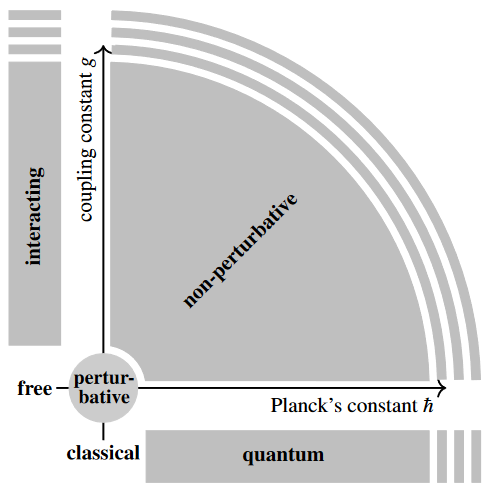Contents

# Contents

## Idea

The construction of quantum field theory is often considered only in the infinitesimal neighbourhood of the classical free field theory; this is called perturbative quantum field theory. Since this very coarse (but remarkably successful) perturbative concept of quantum field theory has come to often be considered by default, one speaks of non-perturbative quantum field theory in order to amplify that the full theory is meant to be considered, not just the perturbative approximation.

More concretely, if we think of generic (Lagrangian) quantum field theories as parameterized by Planck's constant and a given coupling constant, then perturbative QFT concerns just the infinitesimal neighbourhood of the origin in this parameter space, while all the rest of the space is the region of non-perturbative QFT:An important non-perturbative effect in experimentally observed quantum physics that is invisible to perturbative QFT is the confinement and the mass gap of quantum chromodynamics (Yang-Mills theory with simple gauge group).

Presently, non-perturbative quantum field theories have been constructed only in simple toy examples, such as for free field theories (no interaction) or in low spacetime dimension (e.g. 2d CFTs) or for topological quantum field theories.

Indeed the example of non-perturbative quantization of Yang-Mills theory (see there) in 4 dimensions, or even just enough of it to find the expected mass gap of the theory, is listed as one of the open “Millennium Problems” by the Clay Mathematics Institute.

One attempt to construct non-perturbative quantum field theories on Minkowski spacetime was to make rigorous sense of the path integral for the Wick-rotated Euclidean theory by construction of suitable measures. This approach is known as “constructive quantum field theory”. It worked for scalar field theory such as phi^4 theory in spacetime dimension 2 and 3, but (so far) not in dimension 4.

It may be noteworthy that the established construction of perturbative quantum field theory has been understood to be an example of the systematic process of quantization called formal deformation quantization, specifically as an example of Fedosov deformation quantization (Collini 16). Since the non-perturbative version of formal deformation quantization is strict C*-algebraic deformation quantization the latter might be a useful perspective on the problem.

General introduction includes

• Alexander P. Bakulev, Dmitry Shirkov, Inevitability and Importance of Non-Perturbative Elements in Quantum Field Theory, Proceedings of the 6th Mathematical Physics Meeting, Sept. 14–23, 2010, Belgrade, Serbia (ISBN 978-86-82441-30-4), pp. 27–54 (arXiv:1102.2380)

Outlook from the point of view of causal perturbation theory/locally covariant perturbative quantum field theory is in

A textbook account in the school of AQFT is in